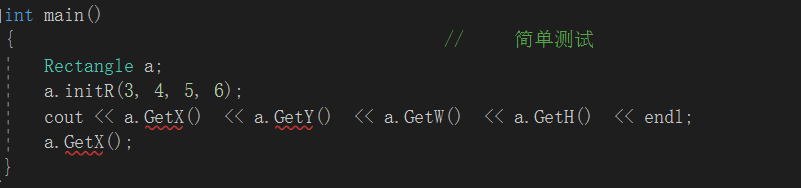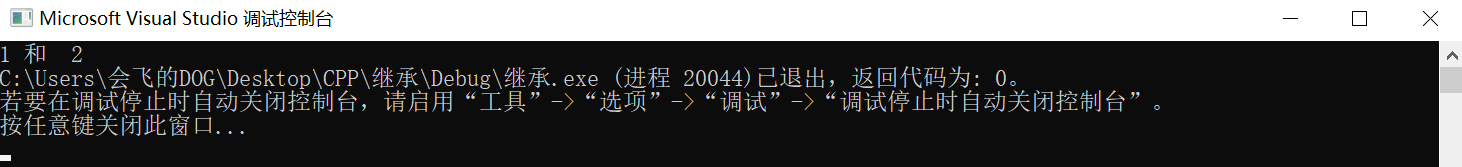# 继承

## 公有继承

### 当继承方式为共有继承时，基类的公有成员和保护成员的访问属性在派生类中不变，而基类的私有成员不可直接访问。可以理解为，在共有继承中，派生类中也具有了基类的公有成员和保护成员，派生类的其他成员可以直接访问，但是基类的私有成员无法访问，只能通过基类的其它成员间接获取。

#include "pch.h"
#include <iostream>
using namespace std;

class Point                                        //  基类的定义
{
public:
Point()
{
}
void initP(float xx, float yy)
{
x = xx;
y = yy;
}

float GetX()
{
return x;

}
float GetY()
{
return y;
}
private:
float x;
float y;
};

class Rectangle : public Point                //     公有继承
{
public:
void initR(float x, float y, float w, float h)
{
initP (x , y);                        //     可以访问到基类的公有和保护成员
W = w;                                //     无法直接对基类私有成员的赋值，只能间接赋值
H = h;
}
float GetH()
{
return H;
}

float GetW()
{
return W;
}

private:                                    //     对派生类进行私有数据成员的添加
float W;
float H;
};

int main()
{                                            //     简单测试
Rectangle a;
a.initR(3, 4, 5, 6);
cout << a.GetX()  << a.GetY()  << a.GetW()  << a.GetH()  << endl;    a.GetX();                               // 通过派生类对象访问基类的成员函数
}

## 私有继承

### 当类的继承方式为私有继承时，基类中的公有成员和保护成员都以私有成员的身份出现在派生类中，而基类的私有成员在派生类不可直接访问。派生类的其他成员可以访问基类的公有和保护成员，但是在类外无法通过派生类的对象访问他们。而对于基类的私有成员，派生类的成员无法直接访问。

class Rectangle : private Point                //     私有继承
{
public:
void initR(float x, float y, float w, float h)
{
initP (x , y);                        //     可以访问到基类的公有和保护成员
W = w;
H = h;
}
float GetH()
{
return H;
}
float GetW()
{
return W;
}
private:
float W;
float H;
};对基类公有成员进行访问，编译器出现语法错误提示。

## 保护继承

### 在保护继承中，基类的公有成员和保护成员都以保护成员的身份出现在基类中，而基类的私有成员不可直接访问。

#include "pch.h"
#include <iostream>
using namespace std;

class Point
{
public:
Point()
{

}
void initP(float xx, float yy)
{
x = xx;
y = yy;
}

float GetX()
{
return x;

}
float GetY()
{
return y;
}
private:
float x;
float y;
};

class Rectangle : protected Point                //     保护继承
{
public:
void initR(float x, float y, float w, float h)
{
initP (x , y);
W = w;
H = h;
}
float GetH()
{
return H;
}

float GetW()
{
return W;
}
private:
float W;
float H;
};

class AA : protected Rectangle                        //   再次利用 保护继承
{
public:
AA(float x, float y, float w, float h){
initR( x, y,  w,  h);
}
void printf()
{
cout << GetX() <<" 和  "<<   GetY();            //此时仍然可以访问到Point的公有函数
}
private:
};

int main()
{                                            //     简单测试
AA a(1, 2, 3, 4);
a.printf();
}在这里，Point类中的公有、保护成员在第一次继承中成为了Rec类中的保护成员，在第二次继承中，同样地以AA类中保护成员的身份存在，所以AA中的成员函数可以访问到。但不用的是，在两次私有继承后，AA中成员函数无法输出结果，因为两次继承中Point类中的成员都以私有成员的身在存在，派生类的对象或者成员都无法访问到。

posted @ 2019-10-12 17:23  会飞的DOG  阅读(233)  评论(0编辑  收藏  举报